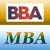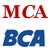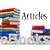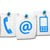# Dynamic Programming

The term 'dynamic programming' refers to a general optimization technique useful for solving a class of multistage problems.

## Dynamic Programming: Definition

Dynamic programming is a methodology useful for solving problems that involve taking decisions over several stages in a sequence.

For instance, consider a company that has to decide on the production plan of' an item for the next three months, so as to meet the demands in different months at minimum cost. The different months for which the production is to be decided, constitute the stages. So it is a multistage problem. These type of problems and a variety of other business problems such as inventory control, replacement, scheduling, capital budgeting, etc., where decisions are made sequentially over several periods can be solved by dynamic programming approach. One thing common to all models in this category is that current decisions influence both present & future periods.

A problem in which the decisions are made at successive stages is called a multistage decision problem.

The dynamic programming approach divides the problem into several sub-problems or stages and then these sub-problems are solved sequentially until the initial problem is finally solved. The common characteristic of all dynamic models is expressing the decision problem by means of a recursive formulation (recursion means determination of a successive element by operations on a preceding element according to a formula).

"In dynamic programming, we creep up on a solution by stages." -Anonymous

•MBA & BBA
•BCA & MCA
•Ebooks & Articles
•Contact us